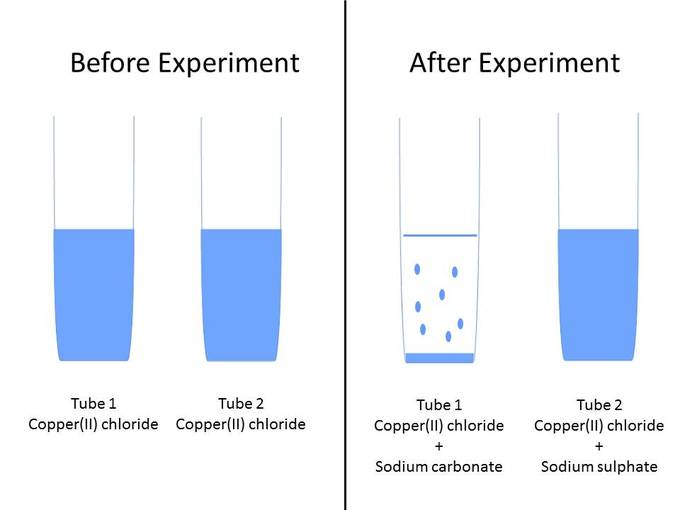## Predicting Precipitation Reactions

#### Learning Objective

• Use the rules of solubility to determine whether a precipitate forms when two solutes are mixed.

#### Key Points

• Sometimes ions in solution react with each other to form a new substance that is insoluble (does not dissolve), called a precipitate.
• A set of rules can be used to predict whether salts will precipitate.
• The property of certain ions to precipitate can be used to isolate a particular ion from the solution.

#### Terms

• cationA positively charged ion, as opposed to an anion.
• anionA negatively charged ion, as opposed to a cation.
• precipitateA solid that exits the liquid phase of a solution.
• precipitateTo come out of a liquid solution into solid form.
• spectator ionAn ion that exists as a reactant and a product in a chemical reaction.
• insolubleThat which cannot be dissolved.

## Precipitation Reactions

Sometimes ions in solution react with each other to form a new substance that precipitates; this reaction is called a precipitation reaction. A precipitate will form if any combination of cations and anions can become a solid.

## Rules of Precipitation

Note that soluble compounds will dissolve in water and insoluble compounds will not.

The following list summarizes the combinations that will form precipitates in solution:

• Nitrates (NO3): All are soluble.
• Potassium (K+), sodium (Na+), and ammonium (NH4+) salts: All are soluble.
• Chlorides (Cl), bromides (Br), and iodides (I): All are soluble except silver (Ag+), lead (II) (Pb2+), and mercury (II) (Hg2+) salts.
• Sulfates (SO42-): All are soluble except lead (II) (Pb2+), barium (Ba2+), and calcium (Ca2+).
• Carbonates (CO32-): All are insoluble except those of potassium (K+), sodium (Na+), and ammonium (NH4+).
• Compounds with fluorine: All are soluble except magnesium (Mg2+), calcium (Ca2+), strontium (Sr2+), barium (Ba2+), and lead(II) (Pb2+).
• Perchlorates (ClO4) and acetates (C2H3O2): All are soluble.
• Metal hydroxides (OH) and oxides (O2-): Most are insoluble.
• Salts of phosphates (PO43-), oxalates (C2O42-), chromates (CrO42-), and sulfides (S2-): Generally insoluble.

## Example of Precipitation Reaction

Imagine you have two test tubes and three solutions: copper (II) chloride (CuCl2) solution, sodium carbonate (Na2CO3) solution, and sodium sulfate (Na2SO4) solution. You put 5 mL of copper (II) chloride into tubes 1 and 2. You add 5 mL of sodium carbonate in tube 1. A precipitate, which looks like light blue specks of dust, forms. You carefully add the sodium sulphate solution into tube 2. No precipitate forms. The solution stays light blue. What happened?Precipitation experimentExample set up for precipitation experiment.

For reaction 1, you have the following ions in your solution: Cu2+, Cl, Na+, and CO32-. The product that forms may be insoluble, in which case a precipitate will form, or soluble, in which case the solution will be clear. Let’s see how the ions in this example could have combined with each other:

$Cu^{2+} + CO_3\ ^{2-} \rightarrow CuCO_3$

$Cu^{2+} + 2Cl^- \rightarrow CuCl_2$

$Na^+ + Cl^- \rightarrow NaCl$

$2Na^+ + CO_3\ ^{2-} \rightarrow Na_2CO_3$

You can automatically exclude the reactions where sodium carbonate and copper (II) chloride are the products because these were the initial reactants. The balanced chemical equation is:

${2Na^+} + {Cu^{2+}} +{CO_3\ ^{2-}} + {Cl^-} \rightarrow {CuCO_3} + {2Na^+} + {2Cl^-}$

You know that sodium chloride (NaCl) is soluble in water, so the remaining product (copper carbonate) must be the one that is insoluble. According to the rules of precipitation, the only soluble carbonates (CO32-) are potassium (K+), sodium (Na+), and ammonium (NH4+). Therefore Na2CO3 will remain in solution, but CuCO3 will precipitate out.

Notice how the sodium and chloride ions remain unchanged during the reaction. They are called spectator ions. They can be removed from the equation yielding the overall precipitation reaction:

$Cu^{2+} + CO_3\ ^{2-} \rightarrow CuCO_3$

Reaction 2 has Cu2+, Cl, Na+, and SO42- in solution. The possible combinations of the ions are as follows:

$Cu^{2+} + SO_4\ ^{2-} \rightarrow CuSO_4$

$Na^+ + Cl^- \rightarrow NaCl$

$Cu^2+ 2Cl^- \rightarrow CuCl_2$

$2Na^+ + SO_4\ ^{2-} \rightarrow Na_2SO_4$

Once again, the reactions where sodium sulfate and copper (II) chloride are the products can be excluded, since they were the initial reactants. The balanced chemical equation is:

${2Na^+} + {SO_4\ ^{2-}} + {Cu^{2+}} + {2Cl^ -} \rightarrow {2Na^+} + {SO_4\ ^{2-}} + {Cu^{2+}} + {2Cl^-}$

If we determine which of these salts are soluble and which are insoluble according to the rules, we see that most chlorides and most sulfates are soluble. This is why no precipitate forms in this second reaction. Even when the ions recombine, they immediately separate and go back into solution.

## Application of Precipitation Reactions

Precipitation reactions are often used to isolate a particular ion from the solution. The process allows for selective removal of ions through properties of solubility. The property is used to separate ions in a method called fractional precipitation.